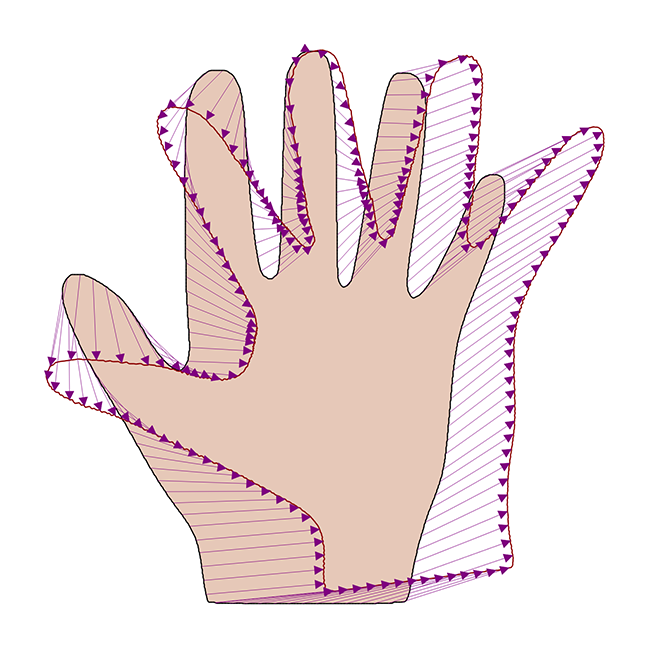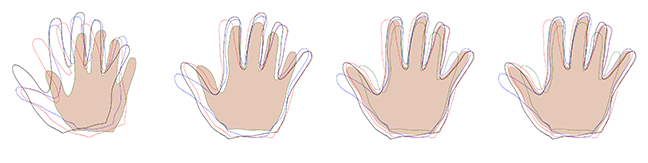## Want to keep learning?

This content is taken from the University of Basel's online course, Statistical Shape Modelling: Computing the Human Anatomy. Join the course to learn more.
2.5

# Superimposing shapes

In the previous video we have seen that if we are given shapes in correspondence, then computing a shape model is straight-forward. However, in our exposition we were a bit careless. Remember that shape is defined only up to translation and rotation. It could still be that the deformation fields contain translation and rotational components instead of pure shape changes.

An example of such a deformation field is shown in Figure 1. Before building the model, we therefore have to correct the pose of the shape. This is usually done using a method called Procrustes alignment, which we will introduce in this article. For the (rather gruesome) origin of the term Procrustes, see this Wikipedia article.Figure 1: a deformation field between two hand shapes, which does not only represent shape changes, but also rotational and translation components.

## Procrustes alignment of two point sets

If two shapes $$\Gamma_R$$ and $$\Gamma_T$$ are in correspondence, this means that for every point $$x \in \Gamma_R$$, we can identify the corresponding point $$y \in \Gamma_T$$. (In the preceding video, we said that the correspondence is induced by the deformation field $$u$$, i.e. $$y = x + u(x)$$.)

Let us pick two finite sets of points on the contour of the shape

$$X = \{x_1, \ldots, x_n\} \subset \Gamma_R$$

and the corresponding points

$$Y = \{y_1, \ldots, y_n\} \subset \Gamma_T.$$

To eliminate the effect of rotation and translation, we can minimise the following optimisation problem

$$\DeclareMathOperator*{\argmin}{arg\,min} \begin{split} (t^*, R^*) = &\argmin_{t \in \mathbb{R}^2, R \in \mathbb{R}^{2 \times 2}} \sum_{i=1}^n \|x_i - R(y_i - t)\|^2, \\ &s.t. R^T R = I, \, \, \det(R)=1 \end{split}$$

to obtain the translation $$t^*$$ and rotation $$R$$, which we can use to superimpose the two shapes.

It turns out that this problem has a closed-form solution both in 2D and 3D, which can be efficiently computed using a singular value decomposition. We refer to the paper by Lorusso et al. for the mathematical details .

## Generalised Procrustes alignment

Given a set of shapes $$\Gamma^{(1)}, \ldots, \Gamma^{(m)}$$, we could pick one of the shapes as a reference shape, say $$\Gamma_R = \Gamma^{(1)}$$, and align all the shapes to this reference using the above procedure. This choice of reference may seem a bit arbitrary. There is, however, a more principled way of doing this, which is usually referred to as generalised Procrustes alignment. The idea is that, since we have correspondence between all the shapes, we can compute a mean shape defined by

$$\Gamma_\mu = \{\mu_1, \ldots, \mu_n\}$$

with

$$\mu_i = \frac{1}{m} \sum_{k=1}^m x_i^{(k)} , \, i =1, \ldots, n.$$

The generalised Procrustes alignment is obtained using the following procedure:

1. Choose an arbitrary shape as the reference shape $$\Gamma_R$$.
2. Align all shapes $$\Gamma^{(1)}, \ldots, \Gamma^{(m)}$$ to $$\Gamma_R$$ using the procedure above.
3. Compute the mean shape $$\Gamma_\mu$$ for the set of aligned shapes.
4. Iterate using the mean shape as the new reference shape.Figure 2: An illustration of the generalised Procrustes alignment.

This procedure is illustrated in Figure 2, where the solid shape indicates the current reference. The leftmost image shows the initial situation, where an arbitrary shape is chosen as a reference. The shapes are aligned to the reference (2nd image) and the mean is recomputed (3rd image). The rightmost image shows the situation after an additional alignment step. We notice that already in this step only minor adjustments are made. Indeed, the procedure usually converges within a few iterations.

 Lorusso, Adele, David W. Eggert, and Robert B. Fisher. A comparison of four algorithms for estimating 3-D rigid transformations. University of Edinburgh, Department of Artificial Intelligence, 1995.